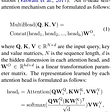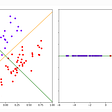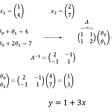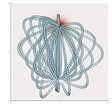# Metric Learning: It’s all about the Distance

Metric Learning = Metric + Learning

We’re sufficiently familiar with both these words. Metric stands for a measure of quantitative assessment, such as a meter for length, and learning refers to the process of acquiring knowledge through study or experience. Interpreting the combination of both these words in the context of Machine Learning, we can define metric learning as the process of learning to define the most appropriate measure of similarity(or differences) over objects under consideration.

Mathematically, we can define a metric as follows:

A metric on a set X is a function (called the distance function or simply distance).

d : X × X R,

where R is a set of real numbers, and for all x,y,z in X following condition are satisfied:

1. d(x, y) 0 (non-negativity)

2. d(x, y) = 0 if and only if x = y (coincidence axiom)

3. d(x, y) = d(y, x) (symmetry)

4. d(x, z) d(x, y) + d(y, z) (triangle inequality).

Here’s how the pipeline for metric learning is different from the one we use for regular ML tasks:

# In a Nutshell-Convex Optimisation Problems

Take a deep breath. Especially if you don’t know what Convex Optimisation Problems are. Or just take one anyway.

Let’s deal with convex functions first. A real-valued function is called a convex function if the line segment joining any two points on the graph always lies on/above the graph.

Here’s to visualize this:

Clearly, convex functions can have only one local minima, which also happens to be the global minima. It’s derivative is forever non-decreasing, and definitely no flat regions in the function’s graph.

Convex Optimisation Problems = Optimisation problems on Convex functions

Naturally, the only optimization you can perform in this case will be minimization.

But why did we jump from metric learning to Convex problems in a flash? Because in a nutshell, metric learning problems are also convex optimization problems. They can be formulated as

`                    min M ℓ(M, S, D, R) + λR(M)`

where ℓ(M, S, D, R) is the loss function, R(M) is some regularizer on the parameters M of the learned metric, and λ ≥ 0 is the regularization parameter.

# Why talk about Metric Learning? That’s not really the end goal, right?

Sure it isn’t. Depending upon the case, the end goal could be coming up with the correct prediction in a classification or regression problem. So why waste time and resources on learning the metric before the trend of the data?

It is imperative that the metric used captures the behavior of the data correctly, which directly impacts the performance of the learning algorithm, and generally proposed distance metrics like Euclidean distance, Manhattan distance, etc often fail to do so. Thus, in the absence of any prior knowledge about the data, we must tune the metric to the data and the problem, deriving the most appropriate measure from the high dimensional data itself. This leads us to invest our efforts into metric learning.

The goal of metric learning is to propose a metric that assigns small distances between similar points and larger distances between dissimilar points.

Fun Fact: Similarity Learning represents a concept similar to that of metric learning, but with much lesser restrictions.

The goal appears to be similar: to learn a similarity function that measures how similar or related two objects are. However, Similarity learning deploys a similarity function, which may or may not qualify as a distance metric.

For instance, often in ranking systems, functions of a specific type known as bilinear functions are used as similarity functions, which may or may not be symmetric. Note that symmetry is one of the 4 criteria required for any similarity function to qualify as a metric, as mentioned above. So bilinear functions that are skew-symmetric can be used for similarity learning but not for metric learning.

# The idea of a Pseudometric

Often, certain distances called pseudo-(meaning ‘false’) metrics make an appearance in metric learning problems, rather than a metric. A pseudometric is a distance that does not follow the coincidence axiom (d(x, y) = 0 if and only if x = y ) but has all other features belonging to a regular metric function. So, a pseudometric allows the distance between two different points to be zero.

Confused how? Let’s take an example.

Consider a function

and a pseudometric

defined on the set of all functions. Clearly, g is

• Non-negative
• Symmetric
• Fulfills the triangle inequality

However, in the case of the coincidence axiom, we find that while

is true, we also have

where clearly g(x,y) is zero despite x and y being distinct.

Pseudometrics had to be defined owing to their natural existence in scenarios such as those of functional analysis and complex manifolds, which are beyond the scope of this article.

# Metric Learning Methods

1. Supervised Metric Learning: Supervised metric learning algorithms take as input points X and target labels y, and learn a distance matrix that makes points from the same class (for classification) or with close target value (for regression) close to each other, and points from different classes or with distant target values far away from each other.

Common supervised metric learning algorithms include:

• Large Margin Nearest Neighbor Metric Learning (LMNN)
• Neighborhood Components Analysis (NCA)
• Local Fisher Discriminant Analysis (LFDA)
• Metric Learning for Kernel Regression (MLKR)

2. Unsupervised Metric Learning: Unsupervised metric learning algorithms only take as input an (unlabeled) dataset X and aim to learn a metric without supervision. A simple baseline algorithm for this task is ‘Covariance’- as the name suggests, it works by calculating the Covariance of the input data.

3. Semi-Supervised Metric Learning: These techniques take as input a small amount of labeled dataset (x,y) combined with a large amount of unlabelled dataset (x) as input for training, making them fall somewhere between supervised and unsupervised methods, as rightly suggested by their name.

4. Weakly Supervised Metric Learning: Weakly supervised algorithms work on weaker information about the data points than supervised algorithms. Rather than labeled points, they take as input similarity judgments on tuples of data points, for instance, pairs of similar and dissimilar points. Note the difference between Semi- and Weakly- Supervised Metric Learning.

Commonly featured algorithms include:

• Information-Theoretic Metric Learning (ITML)
• Sparse High-Dimensional Metric Learning (SDML)
• Relative Components Analysis (RCA)
• Metric Learning with Application for Clustering with Side Information (MMC)

# Applications of metric learning

Technically, any problem that makes use of machine learning algorithms like k-nearest neighbors, k-means, SVMs, etc can deploy metric learning to boost their performance. However, metric and similarity learning most commonly find their use in the following:

1. Recommendation systems (like those used in Netflix, Youtube, etc)

2. Ranking based problems

3. Face identification/verification systems.

4. Patient similarity ( Identification of historical records of patients who are similar to the new patient to help predict clinical outcomes for the new patient).

5. Semantic textual similarity( To determine how similar 2 pieces of texts are), and other such image, video, text, and audio tasks.

Tip for extra reading: Survey/Review papers usually serve as excellent reading material for any topic. They’re essentially research papers that compile the gist of all existing research on a topic in an organized fashion, with some added inputs from the authors. Naturally, they’re long reads but provide a perspective of the development in any field.

For metric learning, I came across the following survey papers and found them useful- Deep Metric Learning: A Survey and A Survey on Metric Learning for Feature Vectors and Structured Data

References:

--

--

--

## More from Vision and Language Group

Vision and Language Group, is a student-run deep learning centric group of IIT Roorkee. We meet up regularly to take up discussions on latest advancements in the deep learning field by reviewing various papers accepted in various tier-1 DL/CV/NLP conferences.

## IBM Research Cracks Code on Accelerating Key Machine Learning Algorithms## Fastformer: Additive Attention Can Be All You Need## Engaging customers through unsupervised and supervised learning## Image Processing with Python: Image Segmentation using Thresholding Methods## Getting Started with AutoML and AWS AutoGluon## Welcoming Deep Learning Weekly to the Comet Community## Integration of Dimension Reduction Methods and Neural Network for Image Classification## Small data, big value## Keerat Kaur Guliani

Research in Applied AI | Machine Intelligence & Deep Learning Enthusiast | Civil Engineering Undergrad @IIT_Roorkee

## Invariances and Symmetries## DEMYSTIFYING THE NAÏVE BAYES CLASSIFIER !!## Regression with Least Squares Intuition## 100-Dimensional Games